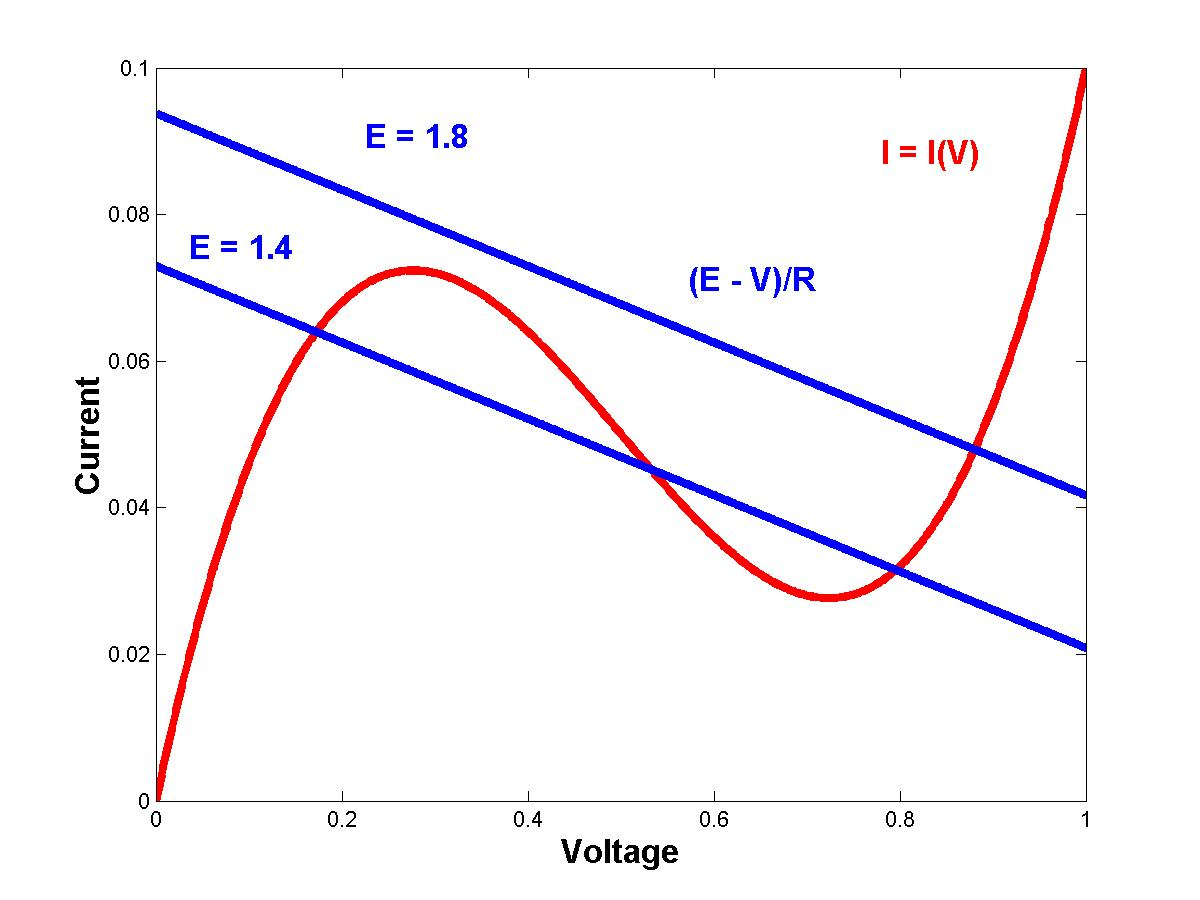## Lecture 1.3:Convergence and stability of iterative methodsTo illustrate the main issues of iterative numerical methods, let us consider the problem of root finding, i.e. finding of possible roots x = x* of a nonlinear equation f(x) = 0. For example, suppose a tunnel diode is supplied by the manufacturer who provides the following voltage V - current I = I(V) characteristic:

I = I(V) = V3 - 1.5*V2 + 0.6*V

The tunnel diode is connected with a resistor R and a voltage source E. Applying the Kirchhoff's voltage law, one can find that the steady current through the diode must satisfy the equation:

I(V) = ( E - V ) / R

For given E and R, this equation is of the form f(x) = 0, where the voltage x = V is to be found. Three versus one solutions are possible (click to enlarge the figure)In order to actually compute these roots, we discuss here three main iterative methods. More methods including globally convergent but slow bracketing methods are also available for root finding (optional chapters 2.2, 2.3, 2.5 of the main textbook).

### Contraction mapping method

Construct the following mapping from xk to xk+1:

xk+1 = g(xk) = xk + f(xk)

Starting with an initial approximation x0, use the iterative scheme above to find x1, x2 etc. If this sequence converges to a fixed point x = x* such that x* = g(x*), then the value x* is the root of the equation f(x) = 0.

The main issue of the iterative method is to check or to prove if the sequence really converges to a fixed point x*. If not, we say that the method diverges. If the method diverges no matter how close the initial approximation x0 to the fixed point x* is, we say that the algorithm is unstable. Otherwise, the algorithm could be stable for smaller errors ek = xk - x* but it could diverges for larger errors ek.

It will be found during the class that the contraction mapping method is linearly stable provided

| g'(x*) | = | 1 + f'(x*) | < 1,

where the prime denotes the derivative of the functions g(x) and f(x). If the method is stable, the absolute error errk reduces with iterations as ek+1 = c ek, where |c| < 1. This is a linear decay (contraction) of the absolute error (the error ek is supposed to be sufficiently small!). According to this linear behavior, we classify the contraction mapping method as a linearly convergent algorithm, or algorithm of order of O(h1), or a first-order algorithm.

### Newton's method

Construct another mapping from xk to xk+1:

xk+1 = xk - f(xk) / f'(xk)

This is the Newton-Raphson method based on the approximation of a function f(x) by the straight line tangent to the curve f(x) at x = xk:

y = f(xk) + f'(xk) (x - xk)

The estimate on the root is obtained by setting y = 0 at x = xk+1. The Newton method is ALWAYS linearly stable (but it may diverge if x0 is sufficiently far from x*)! If f'(x*) is non-zero, the absolute error ek reduces with iterations as ek+1 = c ek2, i.e. at the quadratic rate. Therefore, the Newton's method is fastly convergent (second-order) algorithm or algorithm of order of O(h2). When the root is multiple, i.e. when f'(x*) = 0, the rate of convergence becomes linear again, like in the contraction mapping method.

### Secant method

The slope of the curve f(x) at the point x = xk can be evaluated even if the derivative f'(xk) is too difficult to compute. Indeed, the derivative can be replaced by the quotient between two points [xk,f(xk)] and [xk-1,f(xk-1)]. In this case, the secant method becomes

xk+1 = xk - f(xk) (xk - xk-1) / (f(xk) - f(xk-1))

This approximation when the derivative is replaced by the quotient is called the backward finite difference. We shall study them later in much details.

It is easier to use the secant method compared to the Newton's method. However, the downsides are (i) two starting points x0 and x1 are needed to start iterations and (ii) the rate of convergence is slower than quadratic. The rate is in fact exotic: the absolute error ek decays as ek+1 = c ek1.6918. When the decay power p is between 1 < p < 2, we call the rate of convergence superlinear.Two MATLAB examples illustrate different algorithms for root finding in the problem above. Watch for convergence of the algorithms depending on the initial approximation of a root!

Contraction mapping method
Discusses stability of the method for each of three roots (for a steady current in a tunnel diode)
Newton's method
Discusses the fast (quadratic) rate of convergence for a single root (for the steady current)